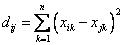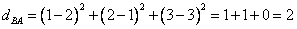## Spearman Distance

Spearman distance is a square of Euclidean distance between two rank vectors.

FormulaExample:

We have ask two persons, A and B about their preference on public transport and here is their ordering vector A = [Bus, Van, Train] and B =[Van, Bus, Train]

Suppose we set [Bus, Van, Train] as pattern-vector we get rank vector A=[1, 2, 3] and B = [2, 1, 3]. Thus, we can represent the two vectors as two points in three-dimensional space. Point A has coordinate (1, 2, 3) and point B has coordinate (2, 1, 3).

The Spearman distance of preference between A and B isPreferable reference for this tutorial is

Teknomo, Kardi (2015) Similarity Measurement. http:\people.revoledu.comkardi tutorialSimilarity